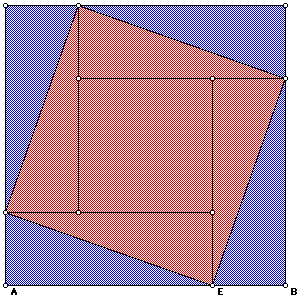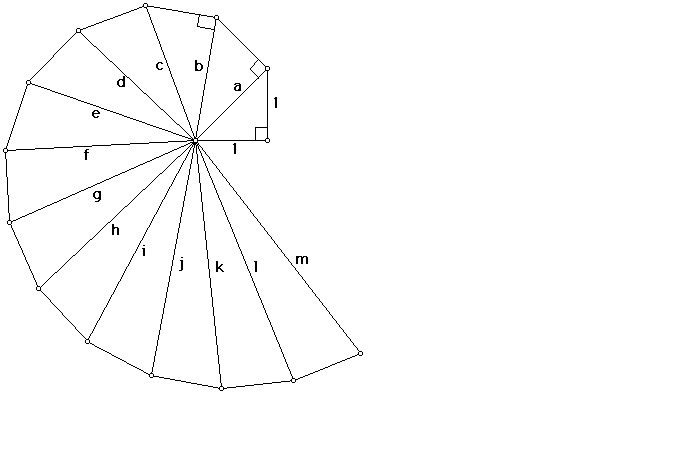# A Brief History of the Pythagorean Theorem

Pythagoras (569-500 B.C.E.) was born on the island of Samos in Greece, and did much traveling through Egypt, learning, among other things, mathematics. Not much more is known of his early years. Pythagoras gained his famous status by founding a group, the Brotherhood of Pythagoreans, which was devoted to the study of mathematics. The group was almost cult-like in that it had symbols, rituals and prayers. In addition, Pythagoras believed that "Number rules the universe,"and the Pythagoreans gave numerical values to many objects and ideas. These numerical values, in turn, were endowed with mystical and spiritual qualities.

Legend has it that upon completion of his famous theorem, Pythagoras sacrificed 100 oxen. Although he is credited with the discovery of the famous theorem, it is not possible to tell if Pythagoras is the actual author. The Pythagoreans wrote many geometric proofs, but it is difficult to ascertain who proved what, as the group wanted to keep their findings secret. Unfortunately, this vow of secrecy prevented an important mathematical idea from being made public. The Pythagoreans had discovered irrational numbers! If we take an isosceles right triangle with legs of measure 1, the hypotenuse will measure sqrt 2. But this number cannot be expressed as a length that can be measured with a ruler divided into fractional parts, and that deeply disturbed the Pythagoreans, who believed that "All is number." They called these numbers "alogon," which means "unutterable." So shocked were the Pythagoreans by these numbers, they put to death a member who dared to mention their existence to the public. It would be 200 years later that the Greek mathematician Eudoxus developed a way to deal with these unutterable numbers.

## The sum of the squares of the sides of a right triangle is equal to the square of the hypotenuse.

This relationship has been known since the days of the ancient Babylonians and Egyptians, although it may not have been stated as explicitly as above. A portion of a 4000 year old Babylonian tablet (c. 1900 B.C.E.), now known as Plimpton 322, (in the collection of Columbia University, New York), lists columns of numbers showing what we now call Pythagorean Triples--sets of numbers that satisfy the equation

a^2 + b^2 = c^2

### Hands On Activity

It is known that the Egyptians used a knotted rope as an aid to constructing right angles in their buildings. The rope had 12 evenly spaced knots, which could be formed into a 3-4-5 right triangle, thus giving an angle of exactly 90 degrees. Can you make a rope like this? Now use your knotted rope to check some right angles in your room at school or at home.

There is further evidence that the Pythagorean relationship was known before the first written proof of it. The pattern of

tiles shown here is typical of those seen in the Orient.

### Can you see the proof of the Pythagorean Theorem in the tile pattern?

If you count the triangles in squares a and b, the legs of the right triangle, you will see that there are 8 in each. The square on the hypotenuse of the triangle, c, contains 16 triangles. It is thought that the Babylonians saw this pattern of tiles to be a proof of the Pythagorean Theorem.

The Chinese used the Pythagorean Theorem as far back as 1000 B.C.E. Can you figure out the method of proof used in thefigure below? Click on the picture for an explanation of the proof.

Euclid, in his book The Elements, presents a proof of the Pythagorean Theorem.

## Excursion

The Pythagoreans discovered, but refused to recognize the existence of, irrational numbers. By starting with an isosceles right triangle with legs of length 1, we can build adjoining right triangles whose hypotenuses are of length sqrt 2, sqrt 3, sqrt 4, sqrt 5, and so on. This construction is sometimes referred to as the Square Root Spiral.### Question to explore:

Can you develop a more direct method of constructing a segment whose length is sqrt 12?

## Beyond the Pythagorean Theorem

In the 17th century, Pierre de Fermat(1601-1665) investigated the following problem: For which values of n are there integral solutions to the equation

x^n + y^n = z^n

We know that the Pythagorean theorem is a case of this equation when n = 2, and that integral solutions exist. Fermat conjectured that there were no solutions when n was greater than 2. He did not leave a proof, though. Instead, in the margin of a textbook, he wrote that he knew that this relationship was not possible, but he did not have enough room on the page to write it down.

His conjecture became known as Fermat's Last Theorem. This may appear to be a simple problem on the surface, but it wasn't until 1993 that Andrew Wiles of Princeton University finally proved the theorem.

